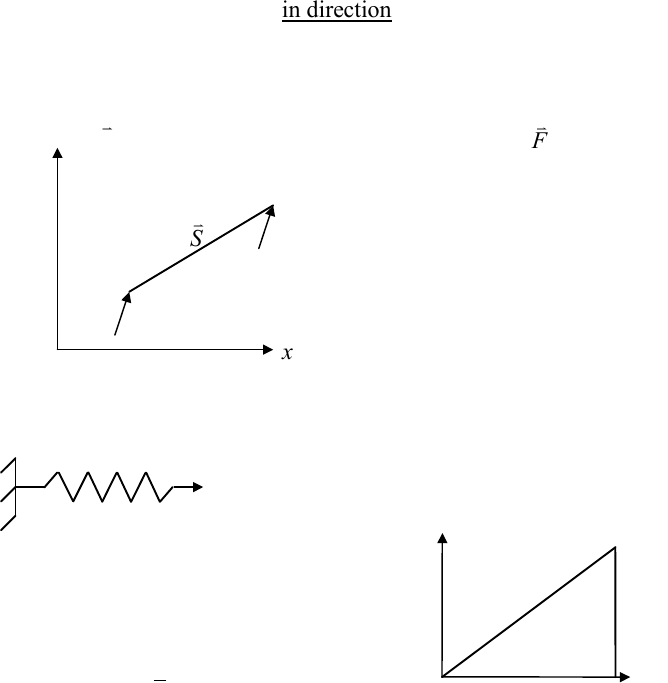Class Notes (1,100,000)
US (480,000)
TAMU (6,000)
AERO (7)
Lecture 20

# AERO 306 Lecture 20: chapter_1

Department
Aerospace Engineering
Course Code
AERO 306
Professor
John Whitcomb
Lecture
20

This preview shows pages 1-3. to view the full 60 pages of the document.
D:\W\Whit\Teaching\_Classes\306\_Notes\1_Intro_VW_Mechanisms\1_intro_virtual_work.docx p. 1 of 16
Chapter 1
Analysis of Mechanisms
Using Virtual Work
What is Virtual Work
&
Why is it useful?

Only pages 1-3 are available for preview. Some parts have been intentionally blurred.

D:\W\Whit\Teaching\_Classes\306\_Notes\1_Intro_VW_Mechanisms\1_intro_virtual_work.docx p. 2 of 16
IntroductiontoVirtualWork
This course relies heavily on something called "Virtual Work" to derive equilibrium
equations. Until now you derived equilibrium equations by imposing the conditions that
the total linear momentum and angular momentum are each zero. When you expressed
the equilibrium equations in terms of forces and moments, you did not consider
kinematics… that is, displacements. That is not to say that you did not eventually express
the forces and moments in terms of displacements through constitutive relations. You had
to whenever you wished to solve any statically indeterminate problem or if you wished to
determine the deformed shape. For virtual work you must consider both forces and
displacements. At first this might seem like an unnecessary complication to have to
consider displacements when deriving the equilibrium equations. Hang in there and you
will soon see that the pain is worth the gain.
Most of the problems you will see in this book can be solved using the concepts that have
been introduced in previous courses. It is logical to ask, “Why are we learning about
something with this unusual name… Virtual Work”. The reason is that the Virtual Work
method forms the basis of the most powerful structural analysis methodology we have
today: finite elements. Using finite elements, we can solve complicated problems that you
cannot begin to solve by trying to solve the differential equations directly. Much of the
course will examine fairly simple problems. This is to minimize the amount of new
material you have to digest while learning the new technique. However, the strategies we
will study are just as valid for solving problems that geniuses could not solve a few
decades ago. You will see later in the course that the primary reason that Virtual Work is
so powerful is because it can easily take advantage of the computational power of
computers.
This new strategy requires the calculation of a special type of work (virtual work). For
there to be work (even virtual work) performed, there must be a force and motion. This
means we must consider both force terms and kinematics (i.e. motion). Before we jump
into Virtual Work, let's first review Real Work

Only pages 1-3 are available for preview. Some parts have been intentionally blurred.D:\W\Whit\Teaching\_Classes\306\_Notes\1_Intro_VW_Mechanisms\1_intro_virtual_work.docx p. 3 of 16
Review of Real Work
work = force * (displacement in direction of force). If the force is variable, we must
integrate
cosWFdSF dS


Examples
y
x
B
A
F
S
If constant, work going from
A to B

F
FS

ˆˆ
Suppose
ˆˆ
Then
F
ai b j
Scidj
Wacbd


NOTE: Work can be negative… if the
force and the displacement are in
opposite directions.
Now let’s consider the deformation of a linear spring.
F
uuo
WFduFku
Wkuduku
u
u

 
0
1
20
2
0
0
0
but
k
F
,u Force vs. Displacement
If we assume the spring is elastic, there is no dissipation, and the work is stored as “strain
energy”. We will not consider inelastic systems, which dissipate energy via friction,
plasticity, and other mechanisms.